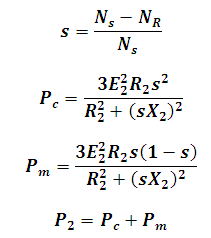# Power in Induction Motor Calculator

This Calctown Calculator calculates the rotor power in an induction motor at a given slip or speed

rpm
rpm
V
ohm
ohm

#### Result

W
W
Wwhere

s = slip

Ns = synchronous speed

Nr = rotor speed

P2 = rotor input power

Pc = rotor copper loss

Pm = mechanical output power

R2 = rotor resistance

X2 = rotor reactance

E2 = rotor emf